# The complete guide to the Disjoint Set Union data structure.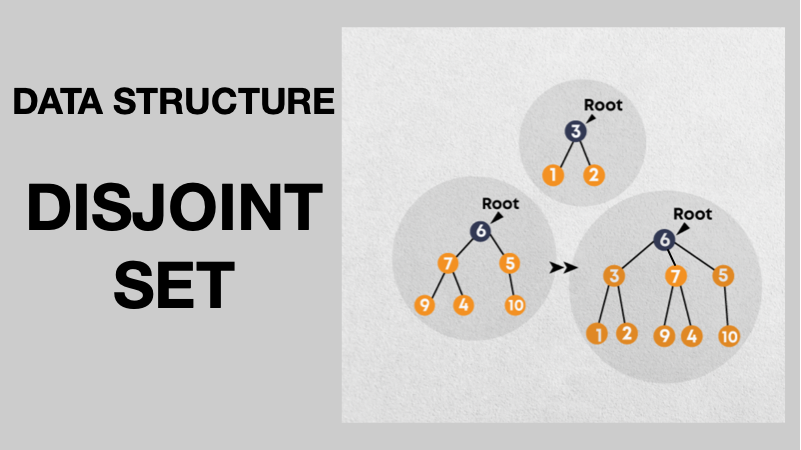A complete introduction to the Disjoint Set Union (DSU) data structure. Read thid post to know what DSU is, how to implement it and when to use it.

The Disjoint Set Union (DSU) is a data structure representing a collection of disjoint sets. It is extremely helpful in solving problems where it is required to track the connectivity of elements belonging to a specific subset and to connect different subsets with each other. Each subset is represented in the form of tree and the root of the tree is the representative element of the set.

The data structure provides the following operations:

• Find(x): find the representative of the set in which the elements x is located
• Unite(x, y): merges the subsets in which the elements x and y are located
• Same(x, y): determines if the elements x and y are located in the same subset

# Data Representation

Instead of representing the subsets using classical trees with nodes and link to child nodes, it is convenient to use an array. Assuming that each of the n elements is associated to an index between 0 and n-1 (e.g. using an hash table), we can use an array of size n to store the index of the parent of each element in the subset. A special case is the representative of each subset which contain its own index. The elements of the array are initialized with their own index, since every element is the representative of its subset before any Unite operation has been performed.

class dsu{

vector<int> parent;

public:

dsu(int n) {
parent.resize(n);
iota(parent.begin(), parent.end(), 0);
}

//public methods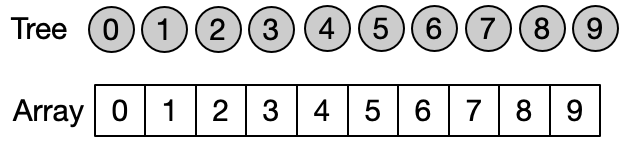# Naive Implementations: quick find and quick union (O(N))

The easiest implementation of DSU assumes that the representative of each element is its parent. This leads to define DSU operations as follows:

• Find(x): return the parent of x
• Unite(x, y): assign x as parent to all elements having y as parent
• Same(x, y): check if x and y have the same parent

This implementation is also known as quick find since the Find operation is performed in constant time. The Unite operation takes instead linear time since every time the entire parents array needs to be traversed.

int find(int x) {
return parents[x];
}

void unite(int x, int y) {

int rootX = find(x);
int rootY = find(y);

for (int& parent : parents) {
if (parent == rootY) {
parent = rootX;
}
}
}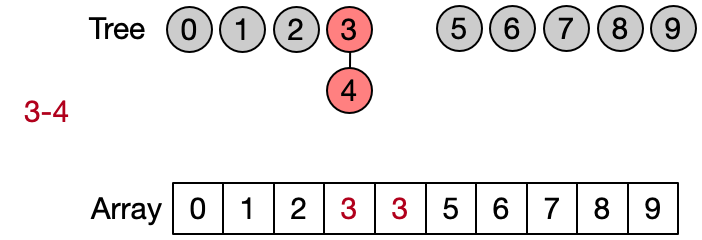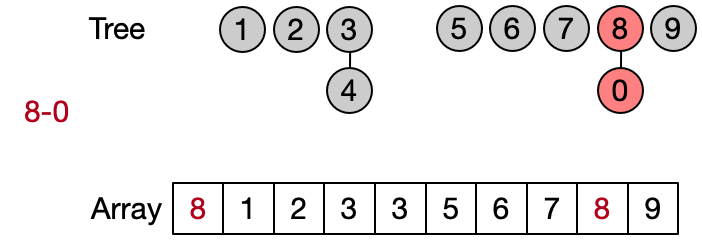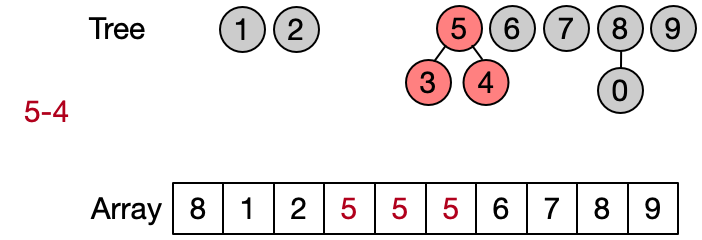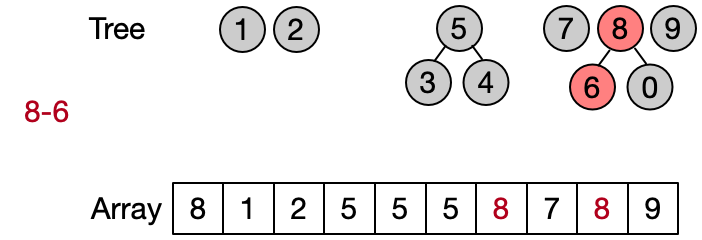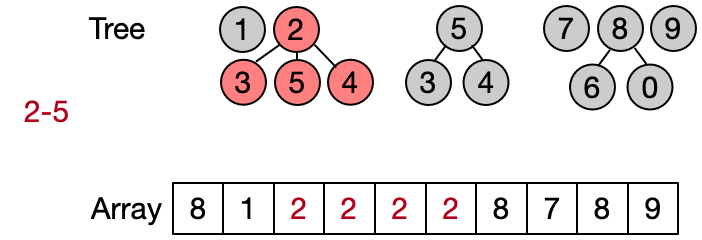An alternative implementation assumes that two elements belong to the same set only if they have the same root. This leads to define DSU operations as follows:

• Find(x): return the root of x
• Unite(x, y): assign root[x] as parent to root[y]
• Same(x, y): check if x and y have the same root

Also in this case the Find operation takes in the worst case linear time because it requires to visit all the ancestors of the given element until the root has not been reached. Anyway, on average the Find performs better because not always the entire parents array needs to be traversed. This implementation is also known as quick union since the Unite operation takes on average less than the one in the quick find.

int find(int x) {

while(parents[x] != x) {
x = parents[x];
}
return x;
}

void unite(int x, int y) {

int rootX = find(x);
int rootY = find(y);

if (rootX != rootY) {
parents[rootY] = rootX;
}
}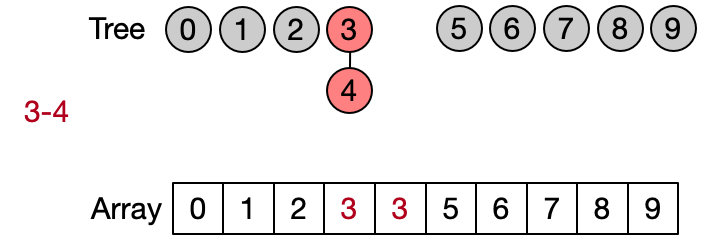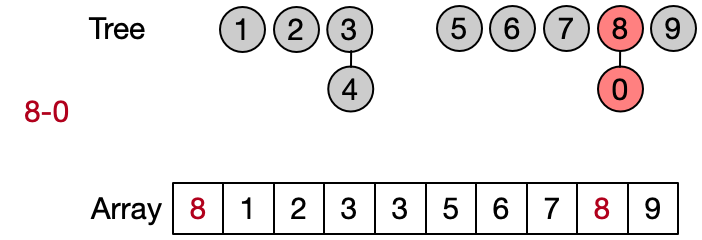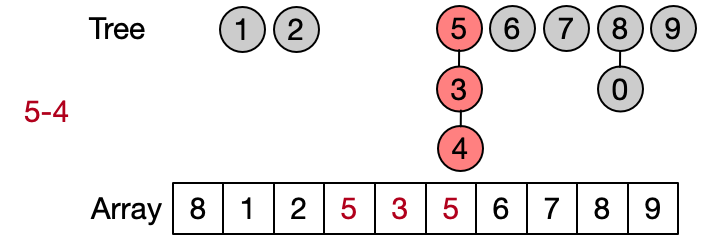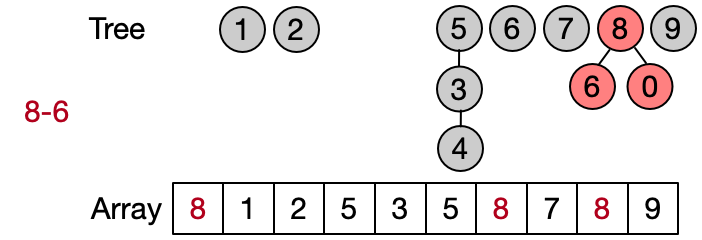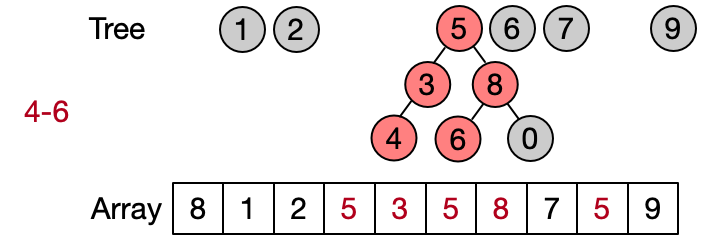# Weighted Union (O(logN))

In the quick union, the Unite operation always attaches the root of the tree of the second element to the root of the tree of the first one. The Weighted Union implementation of the DSU modifies the Unite operation so that the root of the tree having less elements is always attached to the root of the tree having more elements. This simple optimization allows to keep each tree balanced, reducing the time complexity of both the Unite and Find operations to logarithmic. The price to pay for this is the additional space required to keep track of the size of each tree.

void unite(int x, int y) {

int rootX = find(x);
int rootY = find(y);

if (rootX == rootY) return;

if (size[rootX] < size[rootY]) swap(rootX, rootY);
size[rootX] += size[rootY];
parents[rootY] = rootX;
}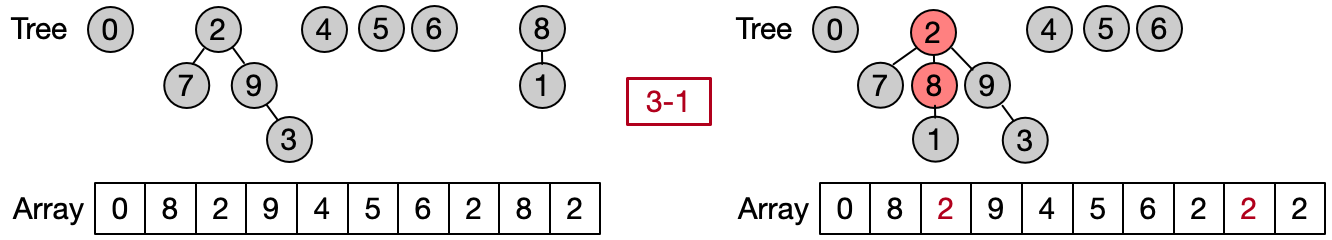# Path Compression (O($\alpha N$))

Path compression is an optimization designed to speed up the Find operation making the path between an element and its root shorter after every execution. There are two main ways to implement such optimization. The first one attaches each element to his grandfather, halving the length of the path between the input element and the root. The second one attaches all the elements on the path directly to the root. The first variant can be easily implemented iteratively, the second one recursively.


//iterative
int find(int x) {

while (parents[x] != x) {
x = parents[x];
parents[x] = parents[parents[x]];
}
return parents[x];
}

//recursive
int find(int x) {

if (parents[x] == x) return x;
return parents[x] = find(parents[x]);
}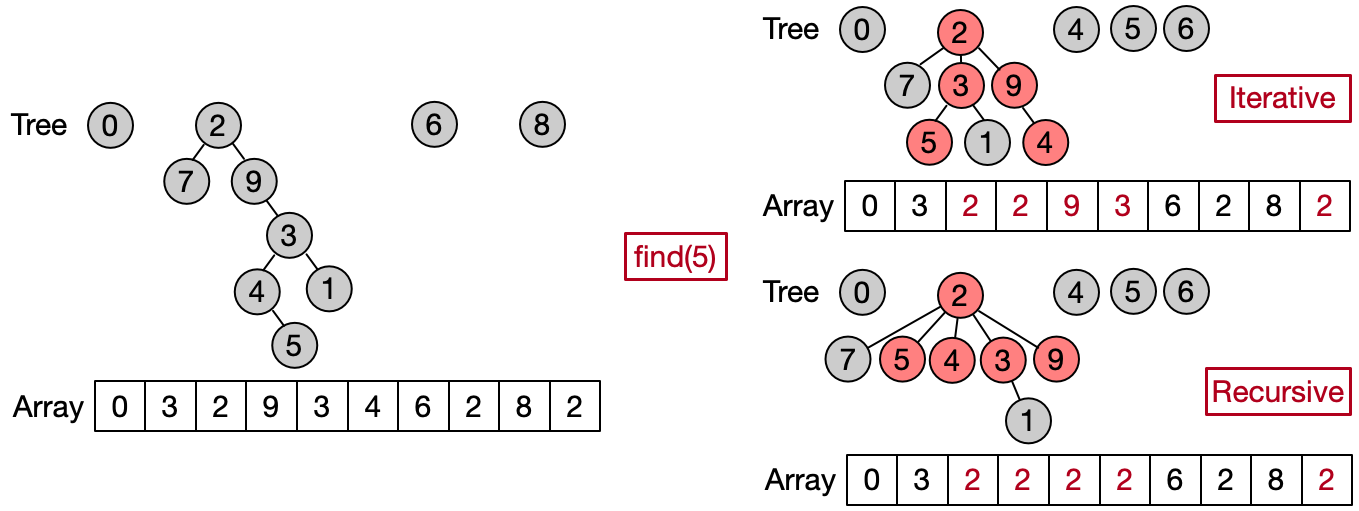Combining path compression with weighted union, we can reduce the time complexity of both the Unite and Find operations nearly to constant. Indeed, it is possible to demonstrate that the final amortized time complexity (i.e. the total time per operation, evaluated over a sequence of multiple operations) is O($\alpha(N)$), where $\alpha(N)$ is the inverse Ackermann function. Such function grows so slowly, that it doesn't exceed 4 for all reasonable values of N.

# Applications

The Disjoint Set Union can significantly reduce the execution time of an algorithm when used in the right context and has many application.

The simpler and most obvious one is to determine the connected components in a graph. For example let's consider the following problem: we are given an unsorted array of N integers (i.e. [9,15,2,6,14,8,4,7]) and we want to compute the length of the longest consecutive elements sequence (i.e. [6,7,8,9] with length 4). The brute force algorithm takes O(N3) time: for each element try to build the longest sequence first on the right and then on the left. A better solution takes O(NlogN) to sort the array and then find the longest sequence with a linear scan.

The DSU solution outperforms both: once we notice that each consecutive sequence can be considered like a connected component, the solution is straightforward. We just need to use an hash table to map number in the sequence to an integer between 0 and N-1 and add an api to the disjoint set data structure to return the size of the largest connected component. You can check the whole code here for more details.

int longestConsecutive(vector<int>& nums) {

unordered_map<int,int> numToPos(nums.size());
for (int i = 0; i < nums.size(); ++i) numToPos[nums[i]] = i;

//initialize parents and weights
dsu dsu(nums.size());

for (int num : nums) {
if (numToPos.count(num-1) > 0) dsu.unite(numToPos[num], numToPos[num-1]);
if (numToPos.count(num+1) > 0) dsu.unite(numToPos[num], numToPos[num+1]);
}

return dsu.maxSize();


Another classical application can be derived from image processing. We are given an image of NxM pixels and we want to determine the size of each connected component having pixels with the same intensity value.

Using DSU, we can do these simply steps:

• iterate over all the pixels in the image;
• for each pixel iterate over its four neighbors;
• perform a Unite operation if the neighbor has the same intensity value.

This approach has also the advantage to process the matrix row by row (i.e. to process a row we only need the previous and the current one) using O(min(N,M)) memory.

However, the most famous application of the DSU is its usage as subroutine of the Kruskal algorithm which finds the minimum spanning tree of a weighted graph. A spanning tree is a subgraph which connects all the vertices and the minimum spanning tree has minimum sum of weights of all its edges. The idea is simple: initially all the vertices are considered as trees isolated from each other and the edges are sorted by weight in non-decreasing order. Then the trees are gradually merged considering an edge at each iteration and combining the two connected trees.

Here the DSU data structure can help to check if the two vertices of each edge are not belonging already to the same tree in order not to introduce a cycle in the spanning tree. The use of DSU enables the algorithm to achieve a time complexity of O(NlogM) instead of O(NlogM + N2), where N is the number of vertices and M the number of edges.


struct Edge {
int u, v, weight;
};

vector<Edge> Kruskal (int n, vector<Edge> const& edges) {

//int cost = 0;
vector<Edge> result;

//initialize parents and weights
dsu dsu(n);

//lambda...
sort(edges.begin(), edges.end(), [](auto const& lhs, auto const& rhs)
{
return lhs.weight < rhs.weight
});

for (Edge e : edges) {
if (dsu.find(e.u) != dsu.find(e.v)) {
//cost += e.weight;
result.push_back(e);
dsu.unite(e.u, e.v);
}
}
}


# Conclusion

The Disjoint Set Union is a fast and simple data structure that every developer should know about since it comes in handy in many application. I hope this article helped you in solidify your knowledge of this data structure and in understanding how the implementation details have here a big impact on the asymptotic performances. You can find in my Github repository a complete implementation of the DSU in multiple programming languages.

If you liked this post, follow me on Twitter to get more related content daily!